Courses

# Fluid Kinematics Civil Engineering (CE) Notes | EduRev

## Civil Engineering (CE) : Fluid Kinematics Civil Engineering (CE) Notes | EduRev

The document Fluid Kinematics Civil Engineering (CE) Notes | EduRev is a part of the Civil Engineering (CE) Course Topic wise GATE Past Year Papers for Civil Engineering.
All you need of Civil Engineering (CE) at this link: Civil Engineering (CE)

Question 1.  The velocity field in a flow system is given by v = 2i+ (x + y)j + (xyz)k. The acceleration of the fluid at (1, 1,2) is    [2019 : 1 Mark, Set-II]
(a) 4i + 12k
(b) 2i + 10k
(c) j + k
(d) 4j + 10k
Answer:
(d)
Solution: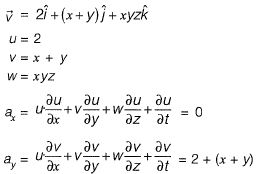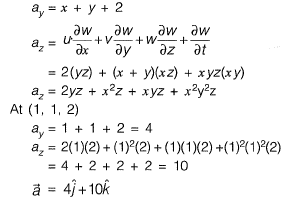Question 2. A flow field is given by u = y2, v = - xy, w = 0.Value of the z-component of the angular velocity (in radians per unit time, up to two decimal places) at the point (0, -1 ,1 ) is _____ .     [2018 : 1 Mark, Set-I]
Solution: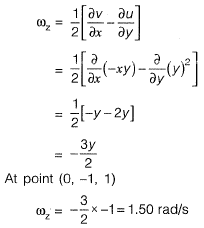Question 3.  The velocity components of a two dimensional plane motion of a fluid are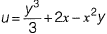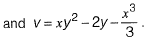The correct statement is:    [2015 : 2 Marks, Set-II]
(a) Fluid is incompressible and flow is irrotational
(b) Fluid is incompressible and flow is rotational
(c) Fluid is compressible and flow is irrotational
(d) Fluid is compressible and flow is rotational
Answer:
(a)
Solution:
Apply continuity equation for 2D in compressible flow,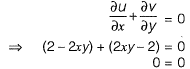Hence, incompressible flow.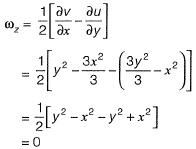Hence, flow is irrotational.

Question 4.  A nozzle is so shaped that the average flow velocity changes linearly from 1.5 m/s at the beginning to 15 m/s at its end in a distance of 0.375 m. The magnitude of the convective acceleration (in m/s2) at the end of the nozzle is _______     [2015 : 1 Mark, Set-II]
Solution:
Convective acceleration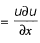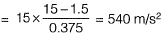Question 5. In a two-dimensional steady flow field, in a certain region of the x-y plane, the velocity component in the x-direction is given by vx = x2 and the density varies as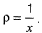Which of the following is a valid expression for the velocity component in the y-direction, vy?
(a)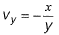(b)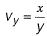(c)  vy = - xy
(d)  vy = x
Answer:
(c)
Solution:
Continuity equation in steady flow,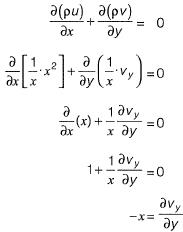On integrating both sides,
vy=-x

Question 6.  A particle moves along a curve whose parametric equations are: x = t3 + 2t, y=-3e-2t and z = 2 sin (5t), where x, y and z show variations of the distance covered by the particle (in cm) with time t(in s). The magnitude of the acceleration of the particle (in cm/s2) at t = 0 is ________ .     [2014 : 2 Marks, Set-I]
Solution: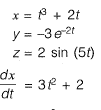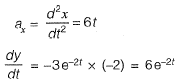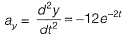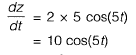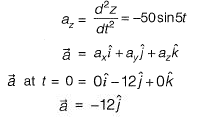⇒Magnitude of acceleration at t =0Question 7. A plane flow has velocity components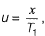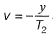and w = 0 along x, y and z directions respectively, where T1 (≠ 0) and T2 (≠ 0) are constants having the dimension of time. The given flow is incompressible if     [2014 : 1 Mark, Set-II]
(a) T1 = - T2
(b)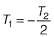(c)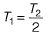(d) T1 = T2
Answer:
(d)
Solution:
For a flow to exist, continuity should be satisfied.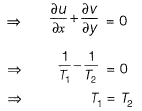Question 8. An incompressible homogeneous fluid is flowing steadily in a variable diameter pipe having the large and small diameters as 15 cm and 5 cm, respectively. If the velocity at a section at the 15 cm diameter portion of the pipe is 2.5 m/s, the velocity of the fluid (in m/s) at a section falling in 5 cm portion of the pipe is _______ .     [2014 : 1 Mark, Set-I]
Solution: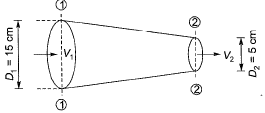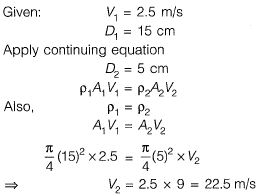Question 9.  For a 2-D flow field, the stream function ψ is given as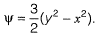The magnitude of discharge occurring between the stream line passing through points (0,3) and (3, 4) is    [2013 : 1 Mark]
(a) 6 units
(b) 3 units
(c) 1.5 units
(d) 2 units
Solution: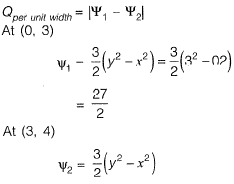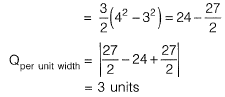Offer running on EduRev: Apply code STAYHOME200 to get INR 200 off on our premium plan EduRev Infinity!

69 docs

,

,

,

,

,

,

,

,

,

,

,

,

,

,

,

,

,

,

,

,

,

;